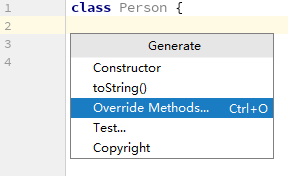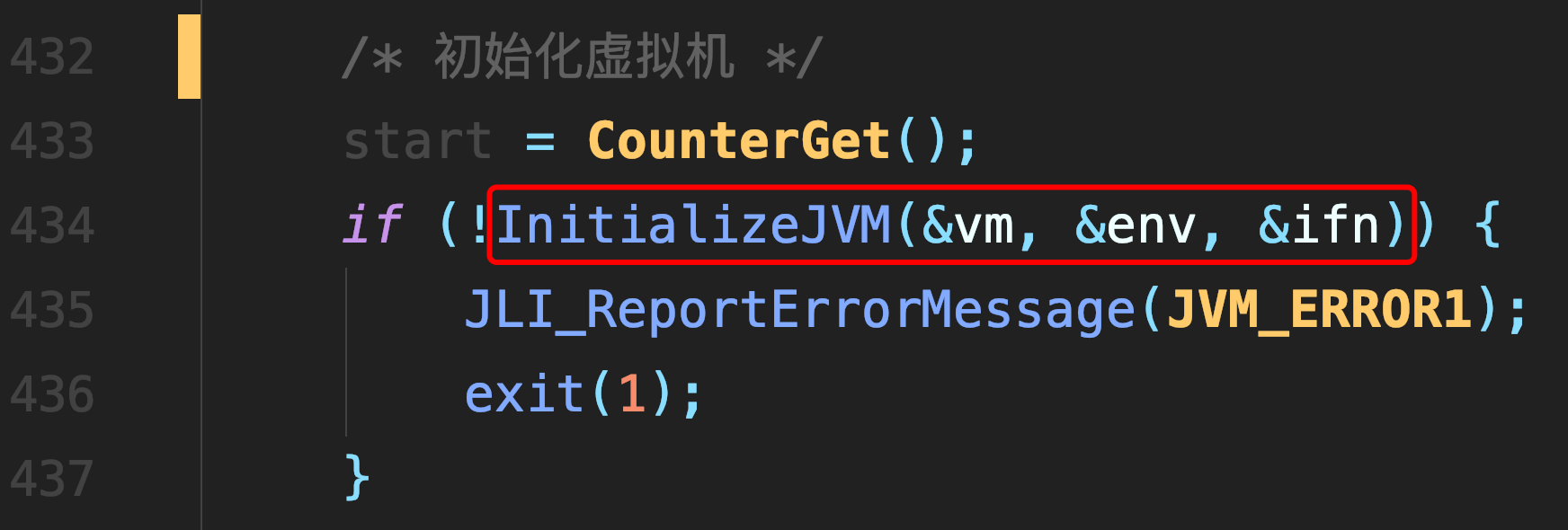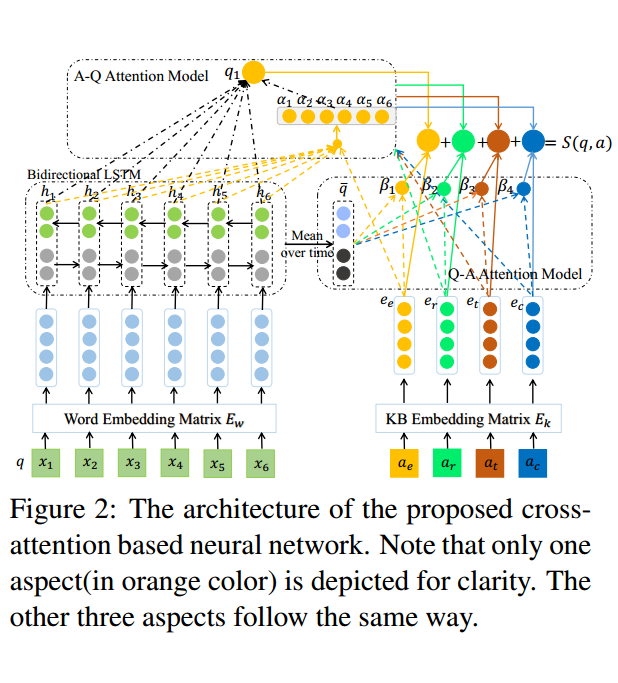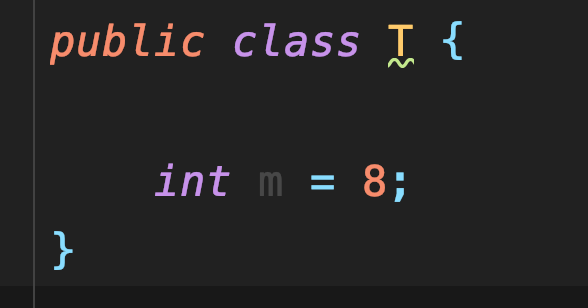# ConcurrentHashMap 核心源码解析——托尔斯泰《安娜?卡列尼娜》

# 1 继承体系

[外链图片转存失败,源站可能有防盗链机制,建议将图片保存下来直接上传(img-gkf7KyhC-1587048420294)(https://user-gold-cdn.xitu.io/2020/4/13/1716f691bd3ef39d “图片标题”)]# 2 属性

• bin数组.第一次插入时才延迟初始化.大小始终是2的幂.由迭代器直接访问.• 下一个要用的 table;仅在扩容时非null• 基本计数器值，主要在没有争用时使用，也用作table初始化竞争期间的反馈.通过CAS更新• table 初始化和扩容的控制
如果为负，则表将被初始化或扩容：
-1用于初始化
-N 活动的扩容线程数
否则，当table为null时，保留创建时要使用的初始表大小，或者默认为0.
初始化后，保留下一个要扩容表的元素计数值.• 扩容时要拆分的下一个表索引（加1）• 扩容和/或创建 CounterCell 时使用的自旋锁（通过CAS锁定）• Table of counter cells。 如果为非null，则大小为2的幂.• Node节点:保存key，value及key的hash值的数据结构,其中value和next都用volatile修饰，保证可见性• 一个特殊的Node节点，转移节点的 hash 值都是 MOVED,-1.其中存储nextTable的引用.在transfer期间插入bin head的节点.只有table发生扩容的时候，ForwardingNode才会发挥作用，作为一个占位符放在table中表示当前节点为null或则已经被移动,# 3 构造方法

## 3.1 无参

• 使用默认的初始表大小（16）创建一个新的空map## 3.2 有参

• 创建一个新的空map，其初始表大小可容纳指定数量的元素，而无需动态调整大小。-创建一个与给定map具有相同映射的新map## tableSizeFor

• 对于给定的所需容量，返回2的幂的表大小## table 的延迟初始化

ConcurrentHashMap在构造函数中只会初始化sizeCtl值，并不会直接初始化table，而是延缓到第一次put操作table初始化.但put是可以并发执行的,是如何保证 table 只初始化一次呢？

``````private final Node<K,V>[] initTable() {
Node<K,V>[] tab; int sc;
// 进入自旋
while ((tab = table) == null || tab.length == 0) {
// 若某线程发现sizeCtl<0，意味着其他线程正在初始化，当前线程让出CPU时间片
if ((sc = sizeCtl) < 0)
else if (U.compareAndSwapInt(this, SIZECTL, sc, -1)) {
try {
// 有可能执行至此时，table 已经非空，所以做双重检验
if ((tab = table) == null || tab.length == 0) {
int n = (sc > 0) ? sc : DEFAULT_CAPACITY;
@SuppressWarnings("unchecked")
Node<K,V>[] nt = (Node<K,V>[])new Node<?,?>[n];
table = tab = nt;
sc = n - (n >>> 2);
}
} finally {
sizeCtl = sc;
}
break;
}
}
return tab;
}
``````

# 4 put

table已经初始化完成，put操作采用CAS+synchronized实现并发插入或更新操作.

``````final V putVal(K key, V value, boolean onlyIfAbsent) {
if (key == null || value == null) throw new NullPointerException();
// 计算hash
int binCount = 0;
// 自旋保证可以新增成功
for (Node<K,V>[] tab = table;;) {
Node<K,V> f; int n, i, fh;
// step1. table 为 null或空时进行初始化
if (tab == null || (n = tab.length) == 0)
tab = initTable();
// step 2. 若当前数组索引无值，直接创建
else if ((f = tabAt(tab, i = (n - 1) & hash)) == null) {
// CAS 在索引 i 处创建新的节点，当索引 i 为 null 时，即能创建成功，结束循环,否则继续自旋
if (casTabAt(tab, i, null,
new Node<K,V>(hash, key, value, null)))
break;                   // no lock when adding to empty bin
}
// step3. 若当前桶为转移节点，表明该桶的点正在扩容，一直等待扩容完成
else if ((fh = f.hash) == MOVED)
tab = helpTransfer(tab, f);
// step4. 当前索引位置有值
else {
V oldVal = null;
// 锁定当前槽点,保证只会有一个线程能对槽点进行修改
synchronized (f) {
// 这里再次判断 i 位置数据有无被修改
// binCount 被赋值，说明走到了修改表的过程
if (tabAt(tab, i) == f) {
// 链表
if (fh >= 0) {
binCount = 1;
for (Node<K,V> e = f;; ++binCount) {
K ek;
// 值有的话，直接返回
if (e.hash == hash &&
((ek = e.key) == key ||
(ek != null && key.equals(ek)))) {
oldVal = e.val;
if (!onlyIfAbsent)
e.val = value;
break;
}
Node<K,V> pred = e;
// 将新增的元素赋值到链表的最后，退出自旋
if ((e = e.next) == null) {
pred.next = new Node<K,V>(hash, key,
value, null);
break;
}
}
}
// 红黑树，这里没有使用 TreeNode,使用的是 TreeBin，TreeNode 只是红黑树的一个节点
// TreeBin 持有红黑树的引用，并且会对其加锁，保证其操作的线程安全
else if (f instanceof TreeBin) {
Node<K,V> p;
binCount = 2;
// 满足if的话，把老的值给oldVal
// 在putTreeVal方法里面，在给红黑树重新着色旋转的时候
// 会锁住红黑树的根节点
if ((p = ((TreeBin<K,V>)f).putTreeVal(hash, key,
value)) != null) {
oldVal = p.val;
if (!onlyIfAbsent)
p.val = value;
}
}
}
}
// binCount不为空，并且 oldVal 有值的情况，说明已新增成功
if (binCount != 0) {
// 链表是否需要转化成红黑树
if (binCount >= TREEIFY_THRESHOLD)
treeifyBin(tab, i);
if (oldVal != null)
return oldVal;
// 槽点已经上锁，只有在红黑树或者链表新增失败的时候
// 才会走到这里，这两者新增都是自旋的，几乎不会失败
break;
}
}
}
// step5. check 容器是否需要扩容，如果需要去扩容，调用 transfer 方法扩容
// 如果已经在扩容中了，check有无完成
return null;
}
``````

## 4.2 执行流程

1. 若数组空，则初始化，完成之后，转2
2. 计算当前桶位是否有值
• 无,则 CAS 创建,失败后继续自旋,直到成功
• 有,转3
3. 判断桶位是否为转移节点(扩容ing)
• 是,则一直自旋等待扩容完成,之后再新增
• 否,转4
4. 桶位有值,对当前桶位加synchronize锁
• 链表，新增节点到链尾
• 红黑树，红黑树版方法新增
5. 新增完成之后,检验是否需要扩容

# 5 transfer - 扩容

``````private final void transfer(Node<K,V>[] tab, Node<K,V>[] nextTab) {
// 旧数组的长度
int n = tab.length, stride;
if ((stride = (NCPU > 1) ? (n >>> 3) / NCPU : n) < MIN_TRANSFER_STRIDE)
stride = MIN_TRANSFER_STRIDE; // subdivide range
// 如果新数组为空，初始化，大小为原数组的两倍，n << 1
if (nextTab == null) {            // initiating
try {
@SuppressWarnings("unchecked")
Node<K,V>[] nt = (Node<K,V>[])new Node<?,?>[n << 1];
nextTab = nt;
} catch (Throwable ex) {      // try to cope with OOME
sizeCtl = Integer.MAX_VALUE;
return;
}
nextTable = nextTab;
transferIndex = n;
}
// 新数组长度
int nextn = nextTab.length;
// 若原数组上是转移节点，说明该节点正在被扩容
ForwardingNode<K,V> fwd = new ForwardingNode<K,V>(nextTab);
boolean finishing = false; // to ensure sweep before committing nextTab
// 自旋，i 值会从原数组的最大值递减到 0
for (int i = 0, bound = 0;;) {
Node<K,V> f; int fh;
int nextIndex, nextBound;
// 结束循环的标志
if (--i >= bound || finishing)
// 已经拷贝完成
else if ((nextIndex = transferIndex) <= 0) {
i = -1;
}
// 每次减少 i 的值
else if (U.compareAndSwapInt
(this, TRANSFERINDEX, nextIndex,
nextBound = (nextIndex > stride ?
nextIndex - stride : 0))) {
bound = nextBound;
i = nextIndex - 1;
}
}
// if 任意条件满足说明拷贝结束了
if (i < 0 || i >= n || i + n >= nextn) {
int sc;
// 拷贝结束，直接赋值，因为每次拷贝完一个节点，都在原数组上放转移节点，所以拷贝完成的节点的数据一定不会再发生变化
// 原数组发现是转移节点，是不会操作的，会一直等待转移节点消失之后在进行操作
// 也就是说数组节点一旦被标记为转移节点，是不会再发生任何变动的，所以不会有任何线程安全的问题
// 所以此处直接赋值，没有任何问题。
if (finishing) {
nextTable = null;
table = nextTab;
sizeCtl = (n << 1) - (n >>> 1);
return;
}
if (U.compareAndSwapInt(this, SIZECTL, sc = sizeCtl, sc - 1)) {
if ((sc - 2) != resizeStamp(n) << RESIZE_STAMP_SHIFT)
return;
i = n; // recheck before commit
}
}
else if ((f = tabAt(tab, i)) == null)
advance = casTabAt(tab, i, null, fwd);
else if ((fh = f.hash) == MOVED)
else {
synchronized (f) {
// 节点的拷贝
if (tabAt(tab, i) == f) {
Node<K,V> ln, hn;
if (fh >= 0) {
int runBit = fh & n;
Node<K,V> lastRun = f;
for (Node<K,V> p = f.next; p != null; p = p.next) {
int b = p.hash & n;
if (b != runBit) {
runBit = b;
lastRun = p;
}
}
if (runBit == 0) {
ln = lastRun;
hn = null;
}
else {
hn = lastRun;
ln = null;
}
// 如果节点只有单个数据，直接拷贝，如果是链表，循环多次组成链表拷贝
for (Node<K,V> p = f; p != lastRun; p = p.next) {
int ph = p.hash; K pk = p.key; V pv = p.val;
if ((ph & n) == 0)
ln = new Node<K,V>(ph, pk, pv, ln);
else
hn = new Node<K,V>(ph, pk, pv, hn);
}
// 在新数组位置上放置拷贝的值
setTabAt(nextTab, i, ln);
setTabAt(nextTab, i + n, hn);
// 在老数组位置上放上 ForwardingNode 节点
// put 时，发现是 ForwardingNode 节点，就不会再动这个节点的数据了
setTabAt(tab, i, fwd);
}
// 红黑树的拷贝
else if (f instanceof TreeBin) {
// 红黑树的拷贝工作，同 HashMap 的内容，代码忽略
...
// 在老数组位置上放上 ForwardingNode 节点
setTabAt(tab, i, fwd);
}
}
}
}
}
}
``````

## 执行流程

1. 首先把原数组的值全部拷贝到扩容之后的新数组，先从数组的队尾开始拷贝
2. 拷贝数组的槽点时，先把原数组槽点锁住，成功拷贝到新数组时，把原数组槽点赋值为转移节点
3. 这时如果有新数据正好需要 put 到该槽点时，发现槽点为转移节点，就会一直等待，所以在扩容完成之前，该槽点对应的数据是不会发生变化的
4. 从数组的尾部拷贝到头部，每拷贝成功一次，就把原数组中的节点设置成转移节点
直到所有数组数据都拷贝到新数组时，直接把新数组整个赋值给数组容器，拷贝完成。

# 6 总结

ConcurrentHashMap 作为一个并发 map,是面试必问点,也是工作中必须掌握的并发容器.

##### 热门推荐
•浏览(604)
•浏览(356)
•浏览(352)
•浏览(345)
•浏览(330)
•浏览(285)
•浏览(284)
•浏览(278)
•浏览(249)
•浏览(249)